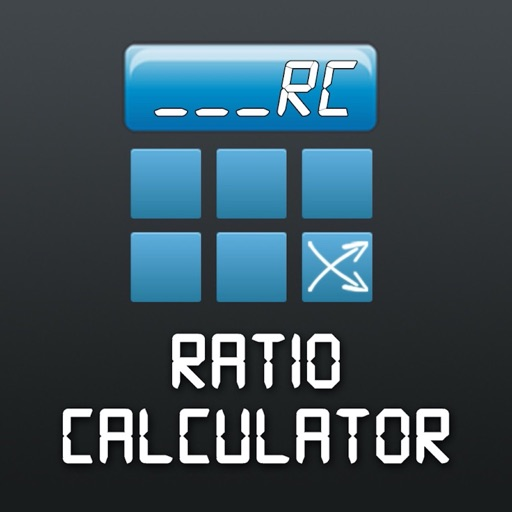Ratio Calculator Tool# Ratio Calculator Tool

Free
0

0 Ratings

Update Date

2021-02-09

Size

21.8 MB

### Screenshots for iPhone

Ratio Calculator Tool Description
Ratio Calculator Tool is not a calculator as we know it. It is designed only for calculating proportions.

It simply gets you the value of [?] in this equation:
"If X Foo equals to Y bla, then Z Foo equals to [?] bla"

It works for 2 ways of proportions: Direct and inverse.

For example when you make a calculation about prices, the result usually increases like this:
If 2 apples cost 10 bucks, 5 apples cost how many bucks?
The app finds 25 bucks here (which is greater than 10).

But when you make a calculation for ex. about speed, the result decreases like this:
If 3 workers finish the job in 15 days, 9 workers will finish in how many days?
The app finds 5 days here (which is less than 15).

Simple, but useful.
Ratio Calculator Tool 1.2.2 Update
2021-02-09 Version History
Thank you for using Ratio Calculator Tool.

This update contains minor improvements.

Stay tuned for more.
More